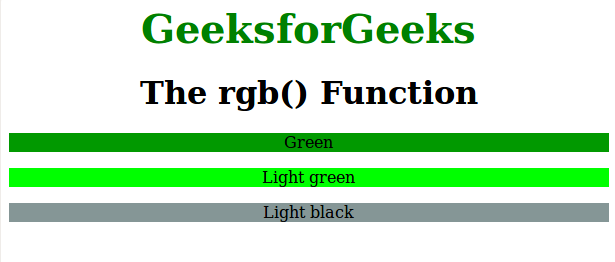Open in App
Not now

# CSS | rgb() Function

• Last Updated : 01 Aug, 2022

The rgb() function is an inbuilt function in CSS which is used to define the colors using the Red Green Blue (RGB) model.

Syntax:

`rgb( red, green, blue )`

Parameters: This function accepts three parameters as mentioned above and described below:

• red: This parameter is used to define the intensity of red color. It is an integer value lies between 0 to 255, or as a percentage value between 0% to 100%.
• green: This parameter is used to define the intensity of green color. It is an integer value lies between 0 to 255, or as a percentage value between 0% to 100%.
• blue: This parameter is used to define the intensity of blue color. It is an integer value lies between 0 to 255, or as a percentage value between 0% to 100%.

Below programs illustrates the rgb() function in CSS:

Program:

## html

 `` `<``html``>` `    ``<``head``>` `        ``<``title``>rgb function` `        ``<``style``> ` `            ``.gfg1 {` `                ``background-color:rgb(1, 153, 0);` `                ``text-align:center;` `            ``}` `            ``.gfg2 {` `                ``background-color:rgb(0, 255, 0);` `                ``text-align:center` `            ``}` `            ``.gfg3 {` `                ``background-color:rgb(133, 150, 150);` `                ``text-align:center` `            ``}` `            ``.gfg {` `                ``font-size:40px;` `                ``font-weight:bold;` `                ``color:green;` `                ``text-align:center;` `            ``}` `            ``h1 {` `                ``text-align:center;` `            ``}` `        ``` `    ``` `    ``<``body``>` `        ``<``div` `class = "gfg">GeeksforGeeks` `        ``<``h1``>The rgb() Function` `        ``<``p` `class = "gfg1">Green` `        ``<``p` `class = "gfg2">Light green` `        ``<``p` `class = "gfg3">Light black` `    ``` `                    `

Output:Supported Browsers: The browser supported by rgb() function are listed below:

• Chrome 1.0 and above
• Edge 12.0 and above
• Internet Explorer 4.0 and above
• Firefox 1.0 and above
• Safari 1.0 and above
• Opera 3.5 and above

My Personal Notes arrow_drop_up
Related Articles